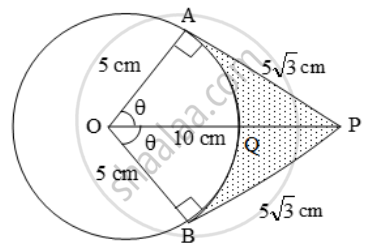# An Elastic Belt is Placed Round the Rim of a Pulley of Radius 5 Cm. One Point on the Belt is Pulled Directly Away from the Centre O of the Pulle - Mathematics

Sum

An elastic belt is placed round the rim of a pulley of radius 5 cm. One point on the belt is pulled directly away from the centre O of the pulley until it is at P, 10 cm from O. Find the length
of the belt that is in contact with the rim of the pulley. Also, find the shaded area.

#### Solution

In the adjacent figure, let ∠AOP = ∠BOP = θ. Clearly, portion AB of the belt is not in contact with the rim of the pulley. In right triangle OAP, we have\cos \theta =\frac{OA}{OP}=\frac{5}{10}=\frac{1}{2}\Rightarrow\theta =60^\text{o}

⇒ ∠AOB = 2θ = 120º

\therefore \text{Arc AB}=\frac{120^\text{o}\times 2\times \pi \times5}{360}=\frac{10\pi }{3}cm[ \text{Using }l=\frac{\theta }{360}\times 2\pi r]

Hence, Length of the belt that is in contact with the rim of the pulley

= Circumference of the rim – Length of arc AB

=\text{ }2\pi \times 5-\frac{10\pi }{3}=\frac{20\pi }{3}cm

Now,

Area of sector OAQB =  \frac { 1 }{ 2 } × π × 5^2 cm^2

=\frac{25\pi }{3}

Area of quadrilateral OAPB = 2 (Area of ∆OAP)

= 2 × (1/2 × OA × AP)

= 5 × 5√3 cm2

[∵ OP^2 = OA^2 + AP^2 ⇒ AP = \sqrt{100-25} = 5√3 ]

= 25√3 cm2

Hence,

OAPB – Area of sector OAQB.

=( 25\sqrt{3}-\frac{25\pi }{3})=\frac{25}{3}(3\sqrt{3}-\pi)

Concept: Circumference of a Circle
Is there an error in this question or solution?

Share